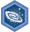## FANDOM

1,895 Pages

Integral calculus: IntegralsDescription
Achieve mastery in all skills in Integral calculus: Integrals
Details
TypeEnergy point(s) awarded 0
Status Available
Disclosed?
Repeating?

This page contains exercises which pertain to the subcategory Integral calculus: Integrals. Mastering them awards user with the Challenge Patch Integral calculus: Integrals as well.

All items (13)

A
•Area between a curve and an axis
•Area under a rate function equals net change
E
•Evaluating a definite integral from a graph
•Evaluating definite integrals
F
•Finding the constant of integration
•Functions defined by integrals
I
•Improper integrals
P
•Properties of integrals
R
•Riemann sums and sigma notation
T
•The definite integral as the limit of a Riemann sum
•The fundamental theorem of calculus
•Trapezoid rule
U
•Using rectangles to approximate area under a curve